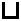Quiz - Cracking Codes
What is Morse Code? *
10 points
In Scratch what is a list? *
10 points
What does this block do? *
10 points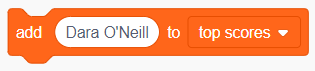What does this block do? *
10 points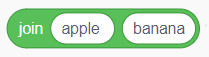What does this block do? *
10 points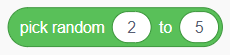How many possible combinations can "secret code" be? *
Tip: take your time with this question and write out all the combinations. You are joining two numbers together.
10 points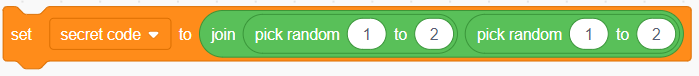When you use an "ask and wait" block in Scratch, where is the answer stored? *
10 points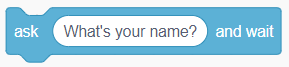Why is a Pigpen Code called a "Pigpen" code? *
10 points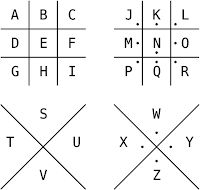What letter does this pigpen code mean? *
Tip: use the Pigpen Code image in the previous question to work it out.
10 points